### Home > CALC > Chapter 1 > Lesson 1.1.1 > Problem1-6

1-6.
1. A car travels 50 miles per hour for 2 hours and 40 miles per hour for 1 hour. Homework Help ✎

1. Sketch a graph of velocity vs. time. Label the axes with units.

2. Fill out the table below for the distance vs. time.

 Time Distance 0.5 1 1.5 2 2.5 3

3. Graph the function of distance vs. time. Label the axes with units.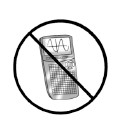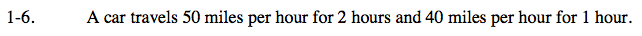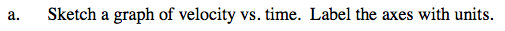Plot time on the x-axis and velocity on the y-axis.

Notice that velocity is constant for the first two hours, and then has a different constant value for the remaining hour. What do constants look like on a graph?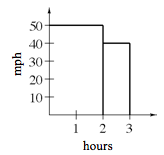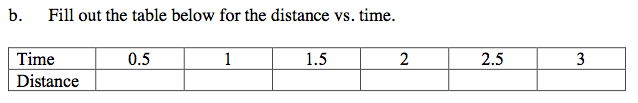Consider the Freeway Fatalities problem 1-1... Recall that distance information can be found by calculating the area under a velocity graph.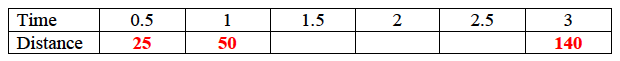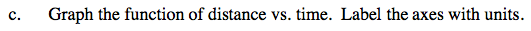Graph the data in the table and connect.
Note that distance is measured in miles and time is measured in hours.